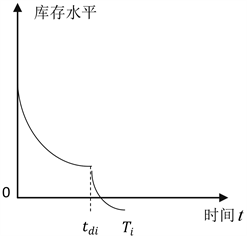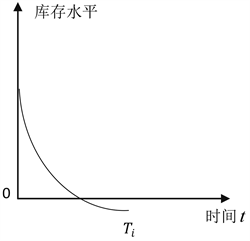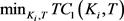# 变质率呈Weibull分布的易腐品联合补货优化Joint Replenishment Optimization of Perishable Products with Metamorphic Rate in Weibull Distribution

• 全文下载: PDF(617KB)    PP.38-46   DOI: 10.12677/MSE.2019.81006
• 下载量: 162  浏览量: 256

This paper studies the exponential distribution of demand obeying in time in an infinite time range. The metamorphic rate is a three-parameter Weibull distribution of multi-variety perishables combined replenishment inventory model, and the goal is to determine the replenishment cycle of each product. The total cost of the retailer is minimized per unit time. The difficulties in this paper are as follows: First, determine the inventory holding cost and deterioration cost during the deterioration of the item; secondly, because the joint replenishment problem is an NP HARD problem, the model is complex and difficult to solve. According to the above difficulties, this paper firstly simplifies the Taylor expansion of the exponential and logarithmic functions existing in the model, and uses the differential evolution algorithm in  to obtain the optimal solution. Retailers for perishables provide useful management advice when implementing joint replenishment.

1. 引言

Ghare和Schrader  最先研究了易变质产品的订货策略，并给出了经典的易腐品库存模型。随后Covert和Philip  松弛了Ghare和Schrader  关于变质率为常数的假设，研究了产品变质率随时间服从Weibull分布的经济订货批量模型。近年来，很多学者对于该模型进行了一系列扩展：在需求率方面，出现了需求率受价格、库存量、时间影响，在变质率方面，出现了变质率服从指数函数，变质率受保存条件影响，以及考虑通货膨胀、价格折扣、商业信用等等的库存模型  -  。

2. 模型构建

2.1. 模型假设与符号表示

1) 不考虑补货提前期，即补货提前期为0。

2) 不允许缺货。

3) 产品i的整体变质率满足三参数的Weibull分布。其中，三参数Weibull分布的概率密度函数、累

4) 由于 $\gamma >0$ ，产品i进入库存内会存在一个固定的非变质期 ${t}_{di}$ ，在 $\left[0,{t}_{di}\right]$ 时段内，产品不发生变质，经过 ${t}_{di}$ 后，产品i的变质率满足 $\theta \left(t\right)=\alpha \beta {t}^{\beta -1}$ 。式中，α、β、γ分别为变质率的尺度因子、形状因子和位

5) 采用周期性查看库存策略，所有产品采用 $\left[T,S\right]$ 补货策略，即存在一个基本补货周期T，每个基本周期对所有产品都进行一次库存检查，产品一个补货成本。每一个产品i在此基础上有一个独立的补货周期  ，且 ${T}_{i}$ 是基本补货周期T的整数( ${k}_{i}$ )倍，所以 ${T}_{i}={k}_{i}T$ 。且在 $i\in \left[1,n\right]$ 中，存在一个 ${k}_{i}=1$ ，且 $T>0$

6) 需求随着时间递增，产品i在t时刻的需求函数可表示为： ${D}_{i}\left(t\right)=A{\text{e}}^{mt}$ ，其中m > 0。

2.2. 数学模型Figure 1. Inventory versus time curve for ${t}_{di}<{T}_{i}$Figure 2. Inventory versus time curve for ${t}_{di}>{T}_{i}$

$\frac{\text{d}{I}_{1i}\left(t\right)}{\text{d}t}=-A{\text{e}}^{mt}$ , $0 (1)

$t=0$ 的时刻，库存水平为 ${Q}_{i}$ ，基于此求解(1)式，可得：

${I}_{1i}\left(t\right)={Q}_{i}-\frac{A}{m}\left({\text{e}}^{mt}-1\right)$ , $0 (2)

$\frac{\text{d}{I}_{2i}\left(t\right)}{\text{d}t}=-A{\text{e}}^{mt}-\theta \left(t\right){I}_{2i}\left(t\right)$ , ${t}_{di} (3)

$t={T}_{i}$ 时间，库存下降为0，基于此求解(3)式，可得：

${I}_{2i}\left(t\right)=A{\text{e}}^{-\alpha {t}^{\beta }}{\int }_{t}^{{T}_{i}}{\text{e}}^{mu+\alpha {u}^{\beta }}\text{d}u$ , ${t}_{di} (4)

$t={t}_{di}$ 时，由函数的连续性可知， ${I}_{1i}\left(t\right)={I}_{2i}\left(t\right)$ ，联合(2)和(4)，可得：

${Q}_{i}=A{\text{e}}^{-\alpha {t}_{di}{}^{\beta }}{\int }_{{t}_{di}}^{{T}_{i}}{\text{e}}^{mu+\alpha {u}^{\beta }}\text{d}u+\frac{A}{m}\left({\text{e}}^{m{t}_{di}}-1\right)$ (5)

${I}_{1i}\left(t\right)=A{\text{e}}^{-\alpha {t}_{di}^{\beta }}{\int }_{{t}_{di}}^{{T}_{i}}{\text{e}}^{mu+\alpha {u}^{\beta }}\text{d}u+\frac{A}{m}\left({\text{e}}^{m{t}_{di}}-{\text{e}}^{mt}\right)$ , $0 (6)

$H{C}_{1}={\sum }_{i=1}^{n}\frac{{h}_{i}}{{T}_{i}}\left[{\int }_{0}^{{t}_{di}}{I}_{1i}\left(t\right)\text{d}t+{\int }_{{t}_{di}}^{{T}_{i}}{I}_{2i}\left(t\right)\text{d}t\right]$ (7)

$D{C}_{1}={\sum }_{i=1}^{n}\frac{{c}_{i}}{{T}_{i}}\left[{I}_{2i}\left({t}_{di}\right)-{\int }_{{t}_{di}}^{{T}_{i}}A{\text{e}}^{mt}\text{d}t\right]$ (8)

$O{C}_{1}=\frac{1}{T}\left(S+{\sum }_{i=1}^{n}\frac{{s}_{i}}{{k}_{i}}\right)$ (9)

$\begin{array}{l}H{C}_{1}=A{\sum }_{i=1}^{n}\frac{{h}_{i}}{{T}_{i}}\left[{t}_{di}{\text{e}}^{-\alpha {t}_{di}^{\beta }}{\int }_{{t}_{di}}^{{T}_{i}}{\text{e}}^{mu+\alpha {u}^{\beta }}\text{d}u+\frac{1}{m}\left({t}_{di}{\text{e}}^{m{t}_{di}}-\frac{1}{m}{\text{e}}^{m{t}_{di}}+\frac{1}{m}\right)\\ \text{\hspace{0.17em}}\text{\hspace{0.17em}}\text{\hspace{0.17em}}\text{\hspace{0.17em}}\text{\hspace{0.17em}}\text{\hspace{0.17em}}\text{\hspace{0.17em}}\text{\hspace{0.17em}}\begin{array}{c}\text{ }\\ \text{ }\end{array}+{\int }_{{t}_{di}}^{{T}_{i}}{\text{e}}^{-\alpha {t}^{\beta }}{\int }_{t}^{{T}_{i}}{\text{e}}^{mu+\alpha {u}^{\beta }}\text{d}u\text{d}t\right]\end{array}$ (10)

 (11)

$T{C}_{1}=H{C}_{1}+D{C}_{1}+O{C}_{1}$

$\begin{array}{c}T{C}_{1}=A\left\{{\sum }_{i=1}^{n}\frac{{h}_{i}}{{T}_{i}}\left[{t}_{di}{\text{e}}^{-\alpha {t}_{di}^{\beta }}{\int }_{{t}_{di}}^{{T}_{i}}{\text{e}}^{mu+\alpha {u}^{\beta }}\text{d}u+\frac{1}{m}\left({t}_{di}{\text{e}}^{m{t}_{di}}-\frac{1}{m}{\text{e}}^{m{t}_{di}}+\frac{1}{m}\right)\\ \text{\hspace{0.17em}}\begin{array}{c}\text{ }\\ \text{ }\end{array}+{\int }_{{t}_{di}}^{{T}_{i}}{\text{e}}^{-\alpha {t}^{\beta }}{\int }_{t}^{{T}_{i}}{\text{e}}^{mu+\alpha {u}^{\beta }}\text{d}u\text{d}t\right]+{\sum }_{i=1}^{n}\frac{{c}_{i}}{{T}_{i}}\left[{\text{e}}^{-\alpha {t}_{di}^{\beta }}{\int }_{{t}_{di}}^{{T}_{i}}{\text{e}}^{mu+\alpha {u}^{\beta }}\text{d}u-\frac{1}{m}\left({\text{e}}^{m{T}_{i}}-{\text{e}}^{m{t}_{di}}\right)\right]\right\}\\ \text{\hspace{0.17em}}\text{\hspace{0.17em}}+\frac{1}{T}\left(S+{\sum }_{i=1}^{n}\frac{{s}_{i}}{{k}_{i}}\right)\end{array}$ (12)

$\frac{\text{d}{I}_{1i}\left(t\right)}{\text{d}t}=-A{\text{e}}^{mt}$ , $0 (13)

${I}_{1i}\left(t\right)=\frac{A}{m}\left({\text{e}}^{m{T}_{i}}-{\text{e}}^{mt}\right)$ (14)

$H{C}_{2}={\int }_{0}^{{T}_{i}}\frac{A}{m}\left({\text{e}}^{m{T}_{i}}-{\text{e}}^{mt}\right)\text{d}t=\frac{A}{m}\left({T}_{i}{\text{e}}^{m{T}_{i}}-\frac{1}{m}{\text{e}}^{m{T}_{i}}+\frac{1}{m}\right)$ (15)

$O{C}_{2}=\frac{1}{T}\left(S+{\sum }_{i=1}^{n}\frac{{s}_{i}}{{k}_{i}}\right)$ (16)

$T{C}_{2}=H{C}_{2}+O{C}_{2}=\frac{A}{m}\left({T}_{i}{\text{e}}^{m{T}_{i}}-\frac{1}{m}{\text{e}}^{m{T}_{i}}+\frac{1}{m}\right)+\frac{1}{T}\left(S+{\sum }_{i=1}^{n}\frac{{s}_{i}}{{k}_{i}}\right)$ (17)

3. 模型求解

3.1. 模型优化

Khouja M, Goyal已经证明JRP问题是NP难题  ，且本模型中的目标函数包含整数和非整数自变量，属于非线性混合整数的分段函数，无法使用常规的非线性规划方法求解。另外，高维度的指数函数使本模型中目标函数的求解变得更加复杂。基于以上原因，本文对目标函数中的指数函数式函数进行泰

$\begin{array}{l}T{C}_{1}\cong \frac{A}{{T}_{i}}{\sum }_{i=1}^{n}\left\{{h}_{i}\left[{t}_{di}\left(1-\alpha {t}_{di}^{\beta }\right){\int }_{{t}_{di}}^{{T}_{i}}1+mu+\alpha {u}^{\beta }\text{d}u+\frac{1}{m}{t}_{di}\left({t}_{di}+2\right)\\ \begin{array}{c}\text{ }\\ \text{ }\end{array}\text{\hspace{0.17em}}\text{\hspace{0.17em}}\text{\hspace{0.17em}}\text{\hspace{0.17em}}\text{\hspace{0.17em}}\text{\hspace{0.17em}}\text{\hspace{0.17em}}\text{\hspace{0.17em}}+{\int }_{{t}_{di}}^{{T}_{i}}\left(1-\alpha {t}^{\beta }\right){\int }_{t}^{{T}_{i}}1+mu+\alpha {u}^{\beta }\text{d}u\text{d}t\right]\\ \text{\hspace{0.17em}}\text{\hspace{0.17em}}\text{\hspace{0.17em}}\text{\hspace{0.17em}}\text{\hspace{0.17em}}\text{\hspace{0.17em}}\text{\hspace{0.17em}}\begin{array}{c}\text{ }\\ \text{ }\end{array}+{c}_{i}\left[\left(1-\alpha {t}_{di}^{\beta }\right){\int }_{t}^{{T}_{i}}1+mu+\alpha {u}^{\beta }\text{d}u-{T}_{i}+{t}_{di}\right]\right\}\frac{1}{T}\left(S+{\sum }_{i=1}^{n}\frac{{s}_{i}}{{k}_{i}}\right)\end{array}$ (18)

(P1):Subject to (18)

3.2. 求解算法设计

3.2.1. 差分进化算法流程

1) 变异操作

2) 交叉操作

2 ${u}_{ij}^{G+1}=\left\{\begin{array}{l}{v}_{ij}^{G+1},\text{\hspace{0.17em}}\text{\hspace{0.17em}}如果\text{ }rand\left(j\right)\le CR\text{ }或\text{ }j=randn\left(i\right)\\ {x}_{ij}^{G},\text{\hspace{0.17em}}\text{\hspace{0.17em}}\text{\hspace{0.17em}}\text{\hspace{0.17em}}\text{\hspace{0.17em}}\text{\hspace{0.17em}}\text{\hspace{0.17em}}\text{\hspace{0.17em}}\text{\hspace{0.17em}}\text{\hspace{0.17em}}\text{\hspace{0.17em}}\text{\hspace{0.17em}}\text{\hspace{0.17em}}\text{\hspace{0.17em}}\text{\hspace{0.17em}}\text{\hspace{0.17em}}\text{\hspace{0.17em}}\text{\hspace{0.17em}}\text{\hspace{0.17em}}否则\end{array}$

$rand\left(j\right)\in \left[0,1\right]$ 是随机选取的一个0~1之间的值，其中j对应着目标向量 ${x}_{ij}^{G}$ 中的第j个维度。 $randn\left(i\right)\in \left[1,2,\cdots ,D\right]$ 为一个随机选择的维数变量。

3) 选择操作

3 ${x}_{i}^{G+1}=\left\{\begin{array}{l}{u}_{i}^{G+1},\text{\hspace{0.17em}}\text{\hspace{0.17em}}\text{\hspace{0.17em}}如果\text{ }f\left({u}_{i}^{G+1}\right)

3.2.2. 本文差分进化算法求解流程

1) 初始化。首先根据本文目标向量的维度及计算能力确定算法的基本控制参数(CR、F、NP)，然后通过随机函数产生初始种群。初始种群中每个解包含变量 ${K}_{i}$ 和T，其中 ${K}_{i}$ 的下限 ${K}_{i}^{LB}$ 为1，由于实际情况分析， ${K}_{i}$ 的上限 ${K}_{i}^{UB}$ 通常小于100，本文中我们取 ${K}_{i}^{UB}=100$ ，在生成初始种群时，  在0和100之间随机取值，然后将随机得到的值取整；经验表明，T在0到1之间随机值。 ${K}_{i}$ 和T共同组成一个 ${x}_{i}$ ，为种群中的一个目标向量。

2) 计算所有的随机产生的目标向量的目标值，得到其成本。

3) 按照上文的方法，种群中的所有目标向量依次进行变异操作、交叉操作，然后对目标向量和实验向量进行选择操作，产生子代种群

4) 将得到的子代种群继续(2)~(3)步骤，直至到达给定的最大迭代次数，算法结束，给出结果。

4. 算例分析及灵敏度分析Table 3. Sensitivity analysis of secondary ordering costsTable 4. α cost sensitivity analysis

5. 结论

  Chen, X., Pang, Z. and Pan, L. (2014) Coordinating Inventory Control and Pricing Strategies for Perishable Products. Operations Research, 62, 284-300. https://doi.org/10.1287/opre.2014.1261  邹广峻, 傅少川. 随机需求下多品种易腐品的联合补货优化[J]. 管理科学与工程, 2018, 7(1): 9-19.  Ghare, P.M. and Schrader, G.H. (1963) A Model for Exponentially Decaying Inventory System. Journal of Industrial Engineering, 14, 238-243.  Covert, R.P. and Philip, G.C. (1973) An EOQ Model for Items with Weibull Distribution Deterioration. AIIE Transaction, 5, 323-326. https://doi.org/10.1080/05695557308974918  Covert, R.P. and Philip, G.C. (1973) An EOQ Model for Items with Weibull Distribution Deterioration. AIIE Transaction, 5, 323-326. https://doi.org/10.1080/05695557308974918  陈六新, 李军, 谢天帅. 价格和库存均影响需求的易腐品模型[J]. 商业研究, 2009(5): 39-43.  文晓巍. 通货膨胀下非常数变质率的易变质商品的EOQ模型[J]. 系统工程, 2011, 29(7): 54-58.  冯海荣, 李军, 曾银莲. 延期支付下的易腐品联合采购费用分配[J]. 系统工程理论与实践, 2013, 33(6): 1411-1423.  王道平, 于俊娣, 李向阳. Weibull变质率和短缺量拖后的易变质物品EOQ模型[J]. 数学的实践与认识, 2011, 41(7): 73-79.  彭扬, 陈金叶, 傅培华. 时变需求下基于部分预付策略的易腐品库存控制[J]. 系统工程, 2016, 34(3): 19-24.  贾涛, 郑毅, 常建龙. 两级商业信用下存在顾客预付的易腐品库存模型[J]. 中国管理科学, 2013, 21(6): 80-87.  冯颖, 蔡小强, 涂菶生, 李勇建. 随机需求情形下单一易变质产品库存模型的订购与定价策略[J]. 南开大学学报(自然科学版), 2010, 43(2): 106-112.  王林, 顿彩霞, 张金隆. 基于混合差分进化算法的联合补货–配送优化模型[J]. 中国管理科学, 2012, 20(3): 86-93.  Khouja, M. and Goyal, S. (2008) A Review of the Joint Replenishment Problem Literature: 1989-2005. European Journal of Operational Research, 186, 1-16. https://doi.org/10.1016/j.ejor.2007.03.007  艾学轶, 张金隆, 徐浩轩, 王林. 需求依赖于变质时间的非立即变质品联合补货优化[J]. 控制与决策, 2018, 33(4): 1-7.  Tsao, Y.C. (2016) Designing a Supply Chain Network for Deteriorating Inventory under Preservation Effort and Trade Credits. International Journal of Production Research, 54, 3837-3851. https://doi.org/10.1080/00207543.2016.1157272  Eynan, A. and Kropp, D.H. (2007) Effective and Simple EOQ-Like So-lutions for Stochastic Demand Periodic Review Systems. European Journal of Operational Research, 180, 1135-1143. https://doi.org/10.1016/j.ejor.2006.05.015  艾学轶, 张金隆, 徐浩轩, 等. 需求依赖于变质时间的非立即变质品联合补货优化[J]. 控制与决策, 2018(4): 671-678.